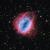# vectors## multiplication of vectors

### cross product

work in progress...## Vector addition

The second question in Sadlers 3C Specialist textbook requires the addition and multiplication of 3 dimensional vectors.

### Addition of vectors

When showing the addition of vectors, we place them end to end. The resulting vector (from the start of the first vector to the end of the last can then be determined.## Vectors in 3 Dimensions

### Mathematical background

In mathematics a vector has a magnitude (length) and direction expressed as an ordered list of tuples (x, y, z). It is sketched as a directed line segment (arrow). Unless otherwise given, a vector does not impart information about location (when studying in secondary education, we use the origin (0,0,0) of a set of axis as the initial point of a vector.